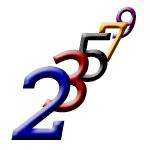# Determine 3948

Determine the quotient and the first member of GP if a3 = 0.52, and a1 + a2 = 0.39.

q1 =  2
q2 =  -0.6667
a1 =  0.13
b1 =  1.17

### Step-by-step explanation:Did you find an error or inaccuracy? Feel free to write us. Thank you!

Tips for related online calculators
Are you looking for help with calculating roots of a quadratic equation?
Do you have a linear equation or system of equations and looking for its solution? Or do you have a quadratic equation?